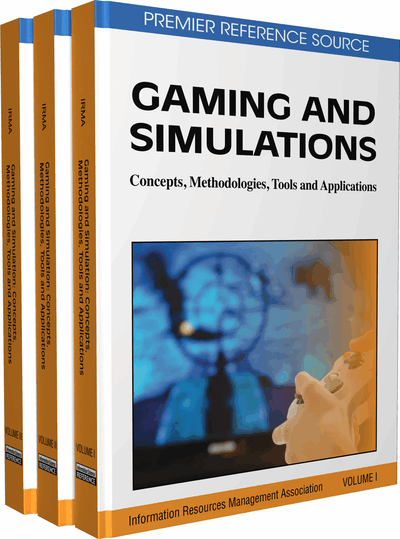# Soft-Constrained Linear Programming Support Vector Regression for Nonlinear Black-Box Systems Identification

Zhao Lu (Tuskegee University, USA) and Jing Sun (University of Michigan, USA)
DOI: 10.4018/978-1-60960-195-9.ch319
Available
\$33.75
List Price: \$37.50
10% Discount:-\$3.75
TOTAL SAVINGS: \$3.75

## Abstract

As an innovative sparse kernel modeling method, support vector regression (SVR) has been regarded as the state-of-the-art technique for regression and approximation. In the support vector regression, Vapnik developed the -insensitive loss function as a trade-off between the robust loss function of Huber and one that enables sparsity within the support vectors. The use of support vector kernel expansion provides us a potential avenue to represent nonlinear dynamical systems and underpin advanced analysis. However, in the standard quadratic programming support vector regression (QP-SVR), its implementation is more computationally expensive and enough model sparsity can not be guaranteed. In an attempt to surmount these drawbacks, this article focus on the application of soft-constrained linear programming support vector regression (LP-SVR) in nonlinear black-box systems identification, and the simulation results demonstrates that the LP-SVR is superior to QP-SVR in model sparsity and computational efficiency
Chapter Preview
Top

## Introduction

Models of dynamical systems are of great importance in almost all fields of science and engineering and specifically in control, signal processing, and information science. A model is always only an approximation of a real phenomenon so that having an approximation theory which allows for the analysis of model quality is a substantial concern. A fundamental principle in system modeling is the Occam’s razor arguing that the model should be no more complex than is required to capture the underlying systems dynamics. This concept, known as the parsimonious principle, which ensures the smallest possible model that explains the data, is particularly relevant in nonlinear model building because the size of a nonlinear model can easily become explosively large.

During the past decade, as an innovative sparse kernel modeling technique, support vector machine (SVM) has been gaining popularity in the field of machine learning and has been regarded as the state-of-the-art technique for regression and classification applications (Cristianini & Shawe-Taylor, 2000; Schölkopf & Smola, 2002; Vapnik, 2000). Essentially, SVM is a universal approach for solving the problems of multidimensional function estimation. Those approaches are all based on the Vapnik-Chervonenkis (VC) theory. Initially, it was designed to solve pattern recognition problem, where in order to find a decision rule with good generalization capability, a small subset of the training data, called the support vectors (SVs), are selected. Experiments showed that it is easy to recognize high-dimensional identities using a small basis constructed from the selected support vectors. Since the inception of this subject, the idea of support vector learning has also been applied to various fields, such as regression, density estimation, and linear operator equation, successfully. When SVM is employed to tackle the problems of function approximation and regression estimation, the approaches are often referred to as the support vector regression (SVR) (Smola & Schölkopf, 2004). The SVR type of function approximation is very effective, especially for the case of having a high-dimensional input space. Another important advantage for using SVR in function approximation is that the number of free parameters in the function approximation scheme is equal to the number of support vectors. Such a number can be obtained by defining the width of a tolerance band, which can be implemented by using the ε-insensitive loss function. Thus, the selection of the number of free parameters can be directly related to the approximation accuracy and does not have to depend on the dimensionality of the input space or other factors as that in the case of multilayer feedforward neural networks.

The ε-insensitive loss function is attractive because unlike the quadratic and Huber cost functions, where all the data points will be support vectors, the SV solution can be sparse. In the realm of data modeling, the sparsity plays a crucial role in improving the generalization performance and computational efficiency. It has been shown that sparse data representations reduce the generalization error as long as the representation is not too sparse, which is consistent with the principle of parsimony (Ancona, Maglietta, & Stella, 2004; Chen, 2006).

## Complete Chapter List

Search this Book:
Reset